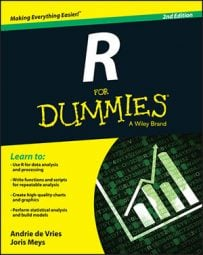##### R For DummiesEach time, you combine a vector with multiple values and one with a single value in a function. R applies the function, using that single value for every value in the vector. But recycling goes far beyond these examples.

Any time you give two vectors with unequal lengths to a recycling function, R repeats the shortest vector as often as necessary to carry out the task you asked it to perform.

Suppose you split up the number of baskets Granny made into two-pointers and three-pointers:

`> Granny.pointers <- c(10, 2, 4, 0, 4, 1, 4, 2, 7, 2, 1, 2)`

You arrange the numbers in such a way that for every game, first the number of two-pointers is given, followed by the number of three-pointers.

Now Granny wants to know how many points she’s actually scored this season. You can calculate that easily with the help of recycling:

```> points <- Granny.pointers * c(2, 3)
> points
 20 6 8 0 8 3 8 6 14 6 2 6
> sum(points)
 87```

Now, what did you do here?

1. You made a vector with the number of points for each basket:

`<span class="code">c(2, 3)</span>`
2. You told R to multiply that vector by the vector Granny.pointers.

R multiplied the first number in Granny.pointers by 2, the second by 3, the third by 2 again, and so on.

3. You put the result in the variable points.

4. You summed all the numbers in points to get the total number of points scored.

In fact, you can just leave out Step 3. The nesting of functions allows you to do this in one line of code:

`> sum(Granny.pointers * c(2, 3))`

Recycling can be a bit tricky. If the length of the longer vector isn’t exactly a multiple of the length of the shorter vector, you can get unexpected results.

Now Granny wants to know how much she improved every game. Being lazy, you have a cunning plan. With diff(), you calculate how many more or fewer baskets Granny made than she made in the game before. Then you use the vectorized division to divide these differences by the number of baskets in the game. To top it off, you multiply by 100 and round the whole vector. All these calculations take one line of code:

```> round(diff(baskets.of.Granny) / baskets.of.Granny * 100 )
1st 2nd 3rd 4th 5th 6th
-67 25 20 50 -67 -267```

That last value doesn’t look right, because it’s impossible to score more than 100 percent fewer baskets. R doesn’t just give you that weird result; it also warns you that the length of diff(baskets.of.Granny) doesn’t fit the length of baskets.of.Granny:

```Warning message:
longer object length is not a multiple of shorter object length```

The vector baskets.of.Granny is six values long, but the outcome of diff(baskets.of.Granny) is only five values long. So the decrease of 267 percent is, in fact, the last value of baskets.of.Granny divided by the first value of diff(baskets.of.Granny). In this example, the shortest vector, diff(baskets.of.Granny), gets recycled by the division operator.

That result wasn’t what you intended. To prevent that outcome, you should use only the first five values of baskets.of.Granny, so the length of both vectors match:

```> round(diff(baskets.of.Granny) / baskets.of.Granny[1:5] * 100)
2nd 3rd 4th 5th 6th
-67 25 20 50 -67```

And all that is vectorization.